Open in App
Not now

# Scala Tutorial – Learn Scala with Step By Step Guide

• Difficulty Level : Medium
• Last Updated : 03 Jan, 2022

Scala is a general-purpose, high-level, multi-paradigm programming language. It is a pure object-oriented programming language which also provides support to the functional programming approach. Scala programs can convert to bytecodes and can run on the JVM(Java Virtual Machine). Scala stands for Scalable language. Scala doesn’t provide any support for .Net Framework. Scala was designed by the Martin Odersky, professor of programming methods at École Polytechnique Fédérale de Lausanne (EPFL) in Switzerland and a German computer scientist. Scala was first released publicly in 2004 on the Java platform as its first version. In June 2004. The latest version of scala is 2.12.6 which released on 27-Apr-2018.Topics:

Features of Scala

Scala has many reasons for being popular and in demand. Few of the reasons are mentioned below:

• Object- Oriented: Every value in Scala is an object so it is a purely object-oriented programming language. The behavior and type of objects are depicted by the classes and traits in Scala.
• Functional: It is also a functional programming language as every function is a value and every value is an object. It provides the support for the high-order functions, nested functions, anonymous functions etc.
• Statically Typed: The process of verifying and enforcing the constraints of types is done at compile time in Scala. Unlike other statically typed programming languages like C++, C etc., Scala doesn’t expect the redundant type of information from the user. In most cases, the user has no need to specify a type.
• Extensible: New language constructs can be added to Scala in form of libraries. Scala is designed to interpolate with the JRE(Java Runtime Environment).
• Concurrent & Synchronize Processing: Scala allows the user to write the codes in an immutable manner that makes it easy to apply the parallelism(Synchronize) and concurrency.

### Application areaGetting Started with Scala

Scala is a very compatible language and thus can very easily be installed into the Windows and the Unix operating systems both very easily.
Since Scala is a lot similar to other widely used languages syntactically, it is easier to code and learn in Scala. scala programs can be written on any plain text editor like notepad, notepad++, or anything of that sort. One can also use an online IDE for writing Scala codes or can even install one on their system to make it more feasible to write these codes because IDEs provide a lot of features like intuitive code editor, debugger, compiler, etc.
To begin with, writing Scala Codes and performing various intriguing and useful operations, one must have scala installed on their system. This can be done by following the step by step instructions provided below:

1. Verifying Java Packages
The first thing we need to have is a Java Software Development Kit(SDK) installed on the computer. We need to verify this SDK packages and if not installed then install them.
2. Now install Scala
3. Testing and Running the Scala Commands
Open the command prompt now and type in the following codes.

`C:\Users\Your_PC_username>scala`
1. We will receive an output as shown below: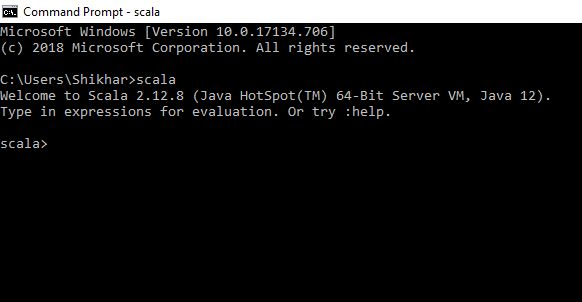1. Output of the command.

How to Run a Scala Program ?

Let’s consider a simple Hello World Program.

## Scala

 `// Scala program to print Hello World` `// Creating object``object` `Geeks {` `// Main method``def` `main(args``:` `Array[String])``{``    ` `    ``// prints Hello, Geeks!``    ``println(``"Hello, World!"``)``}``} `

Output:

`Hello, World!`

Generally, there are two ways to Run a Scala program-

• Using Online IDEs: We can use various online IDEs which can be used to run Scala programs without installing.
• Using Command-Line: We can also use command line options to run a Scala program. Below steps demonstrate how to run a Scala program on Command line in Windows/Unix Operating System:

Open Commandline and then to compile the code type scala Hello.scala. If your code has no error then it will execute properly and output will be displayed.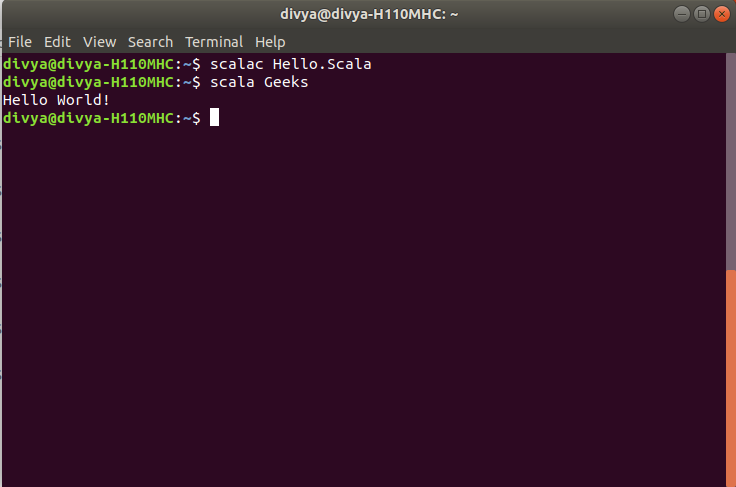Fundamentals of Scala

Variables

Variables are simply a storage location. Every variable is known by its name and stores some known and unknown piece of information known as value. In Scala there are two types of variable:

• Mutable Variables: These variables are those variables which allow us to change a value after the declaration of a variable. Mutable variables are defined by using the “var” keyword.
• Immutable Variables: These variables are those variables which do not allow you to change a value after the declaration of a variable. Immutable variables are defined by using the “val” keyword.

Example:

```// Mutable Variable
var name: String = "geekforgeeks";

// Immutable Variable
val name: String = "geekforgeeks";```

To know more about Scala Variables refer – Variables in Scala, Scope of Variable in Scala

Operators

An operator is a symbol that represents an operation to be performed with one or more operand. Operators allow us to perform different kinds of operations on operands. There are different types of operators used in Scala as follows:

Example :

## Scala

 `// Scala program to demonstrate``// the Operators` `// Creating object``object` `Geeks``{``    ` `    ``// Main method``    ``def` `main(args``:` `Array[String])``    ``{``    ` `        ` `        ``// Operands``        ``var` `a ``=` `10``;``        ``var` `b ``=` `4``;``        ``var` `c ``=` `true``;``        ``var` `d ``=` `false``;``        ``var` `result ``=` `0``;``           ` `        ``// using arithmetic operators ``        ``println (``"Addition is: "``+ (a + b) );``        ``println (``"Subtraction is: "``+ (a - b) ) ;``         ` `        ``// using Relational Operators``        ``if` `(a ``==` `b)``        ``{``           ``println (``"Equal To Operator is True"``);``        ``} ``        ``else``        ``{``           ``println (``"Equal To Operator is False"``);``        ``}``         ` `        ``// using Logical Operator 'OR'``        ``println(``"Logical Or of a || b = "` `+ (c || d)); ``         ` `        ``// using Bitwise AND Operator``        ``result ``=` `a & b;``        ``println (``"Bitwise AND: "` `+ result );``         ` `        ``// using Assignment Operators``        ``println (``"Addition Assignment Operator: "` `+ (a +``=` `b) );``        ` `    ``}``}`

Output:

```Addition is: 14
Subtraction is: 6
Equal To Operator is False
Logical Or of a || b = true
Bitwise AND: 0

Decision Making

Decision Making in programming is similar to decision making in real life. Scala uses control statements to control the flow of execution of the program based on certain conditions. These are used to cause the flow of execution to advance and branch based on changes to the state of a program.

#### Decision Making Statements in Scala :

• If
• If – else
• Nested – If

Example 1: To illustrate use of if and if-else

## Scala

 `// Scala program to illustrate the if and if-else statement``object` `Test``{``    ` `// Main Method``def` `main(args``:` `Array[String])``{``    ` `    ``// taking a variable``    ``var` `a``:` `Int ``=` `650``        ` `    ``// if condition to check``    ``// for even number``    ``if``(a ``%` `2` `==` `0``)``    ``{``        ``println(``"Even Number"``)``    ``}``    ` `    ``if` `(a ``>` `698``)``    ``{` `        ``// This statement will not``        ``// execute as a > 698 is false``        ``println(``"GeeksforGeeks"``)``    ``}``    ` `    ``else``    ``{``        ` `        ``// This statement will execute``        ``println(``"Sudo Placement"``)``    ``}``}``}`

Output:

```Even Number
Sudo Placement```

Example 2: To illustrate the use of Nested-if

## Scala

 `// Scala program to illustrate ``// Nested if statement``object` `Test``{``     ` `// Main Method``def` `main(args``:` `Array[String])``{   ``    ``var` `a``:` `Int ``=` `10``;``         ` `    ``if``(a ``%` `2` `==` `0``)``    ``{ ``        ``// Nested - if statement``        ``// Will only be executed ``        ``// if above if statement``        ``// is true``        ``if``(a ``%` `5` `==` `0``)``        ``{  ``            ``println(``"Number is divisible by 2 and 5\n"``)  ``        ``} ``    ``}``}``}`

Output:

`Number is divisible by 2 and 5`

To know more about Decision Making please refer to Decision making in Scala

Loops

Looping in programming languages is a feature which facilitates the execution of a set of instructions/functions repeatedly while some condition evaluates to true. Loops make the programmer’s task simpler. Scala provides the different types of loop to handle the condition based situation in the program. The loops in Scala are :

## Scala

 `// Scala program to illustrate for loop``object` `forloopDemo {``      ` `   ``// Main Method``   ``def` `main(args``:` `Array[String]) {``         ` `      ``var` `y ``=` `0``;``        ` `      ``// for loop execution with range``      ``for``(y ``<``- ``1` `to ``4``)``      ``{``         ``println(``"Value of y is: "` `+ y);``      ``}``   ``}``}`

•
Output:

```Value of y is: 1
Value of y is: 2
Value of y is: 3
Value of y is: 4```

## Scala

 `// Scala program to illustrate while loop``object` `whileLoopDemo``{``      ` `    ``// Main method``    ``def` `main(args``:` `Array[String])``    ``{``        ``var` `x ``=` `1``;``  ` `        ``// Exit when x becomes greater than 4``        ``while` `(x ``<=` `4``)``        ``{``            ``println(``"Value of x: "` `+ x);``  ` `            ``// Increment the value of x for``            ``// next iteration``            ``x ``=` `x + ``1``;``        ``}``    ``}``}`

•
Output:

```Value of x: 1
Value of x: 2
Value of x: 3
Value of x: 4```

## Scala

 `// Scala program to illustrate do..while loop``object` `dowhileLoopDemo``{``      ` `    ``// Main method``    ``def` `main(args``:` `Array[String])``    ``{``        ``var` `a ``=` `10``;``  ` `        ``// using do..while loop``        ``do``        ``{``            ``print(a + ``" "``);``            ``a ``=` `a - ``1``;``        ``}``while``(a ``>` `0``);``    ``}``}`

•
Output:

`10 9 8 7 6 5 4 3 2 1 `

•

Arrays

Array is a special kind of collection in scala. it is a fixed size data structure that stores elements of the same data type. It is a collection of mutable values. Below is the syntax.
Syntax :

`var arrayname = new Array[datatype](size)`It will create an array of integers which contains the value 40, 55, 63, 17 and many more. Below is the syntax to access a single element of an array, if we’ve created an array named number.

`number(0)`

It will produce the output as 40.
Iterating through an Array:

In this example we create an array while providing the values of its elements at the same time. In this case, the type is inferred.

## Scala

 `// Scala program to accessing an array ``// of the string as name.``object` `GFG``{``    ``// Main method``    ``def` `main(args``:` `Array[String]) ``    ``{``        ``// allocating memory of 1D Array of string. ``        ``var` `name ``=` `Array(``"gfg"``, ``"geeks"``, ``"GeeksQuize"``, ``                    ``"geeksforgeeks"` `)``      ` `        ``println(``"second element of an array is: "``)``          ` `        ``// Accessing an array element``        ``println(name(``1``) )``    ``}``}`

Output:

```second element of an array is:
geeks```

Strings

A string is a sequence of characters. In Scala, objects of String are immutable which means a constant and cannot be changed once created. In Scala a String type is specified before meeting the string literal. but when the compiler meet to a string literal and creates a string object str.
Syntax :

```var str = "Hello! GFG"
or
val str = "Hello! GFG"

var str: String = "Hello! GFG"
or
val str: String = "Hello! GFG"```

## Scala

 `// Scala program to illustrate how to ``// create a string``object` `Main``{``      ` `    ``// str1 and str2 are two different strings``    ``var` `str``1` `=` `"Hello! GFG"``    ``val` `str``2``:` `String ``=` `"GeeksforGeeks"``    ``def` `main(args``:` `Array[String]) ``    ``{``          ` `        ``// Display both strings``        ``println(str``1``);``        ``println(str``2``);``    ``}``}`

Output:

```Hello! GFG
GeeksforGeeks```

#### Concatenating strings in Scala:

when a new string is created by adding two strings is known as a concatenation of strings. Scala provides concat() method to concatenate two strings, this method returns a new string which is created using two strings. You can also use ‘+’ operator to concatenate two strings.

## Scala

 `// Scala program to illustrate how to ``// concatenate strings``object` `Main ``{``      ` `    ``// str1 and str2 are two strings``    ``var` `str``1` `=` `"Welcome! GeeksforGeeks "``    ``var` `str``2` `=` `" to Portal"``      ` `    ``// Main function``    ``def` `main(args``:` `Array[String])``    ``{``          ` `        ``// concatenate str1 and str2 strings``        ``// using concat() function``        ``var` `Newstr ``=` `str``1``.concat(str``2``);``          ` `        ``// Display strings ``        ``println(``"String 1:"` `+str``1``);``        ``println(``"String 2:"` `+str``2``);``        ``println(``"New String :"` `+Newstr);``          ` `        ``// Concatenate strings using '+' operator``        ``println(``"This is the tutorial"` `+ ``                    ``" of Scala language"` `+ ``                    ``" on GFG portal"``);``    ``}``}`

Output:

```String 1:Welcome! GeeksforGeeks
String 2: to Portal
New String :Welcome! GeeksforGeeks  to Portal
This is the tutorial of Scala language on GFG portal```

Functions

A function is a collection of statements that perform a certain task. Scala is assumed as functional programming language so these play an important role. It makes easier to debug and modify the code. Scala functions are first class values. Below is the syntax of Scala Functions.
Syntax:

```def function_name ([parameter_list]) : [return_type] = {

// function body

}```

def keyword is used to declare a function in Scala.
Function Calling : There are mainly two ways to call the function in Scala. First way is the standard way as follows:

`function_name(paramter_list)`

In the Second way, a user can also call the function with the help of the instance and dot notation as follows:

`[instance].function_name(paramter_list)`

## Scala

 `// Scala program of function calling``object` `GeeksforGeeks {``      ` `   ``def` `main(args``:` `Array[String]) {``         ` `      ``// Calling the function``      ``println(``"Sum is: "` `+ functionToAdd(``5``, ``3``));``   ``}``     ` `     ` `   ``// declaration and definition of function``   ``def` `functionToAdd(a``:``Int, b``:``Int) ``:` `Int ``=``   ``{``         ` `       ``var` `sum``:``Int ``=` `0``       ``sum ``=` `a + b``  ` `       ``// returning the value of sum``       ``return` `sum``   ``}``}`

Output :

`Sum is: 8`

Anonymous Functions in Scala :
In Scala, An anonymous function is also known as a function literal. A function which does not contain a name is known as an anonymous function.
Syntax :

```(z:Int, y:Int)=> z*y
Or
(_:Int)*(_Int)```

## Scala

 `// Scala program to illustrate the anonymous method``object` `Main ``{``    ``def` `main(args``:` `Array[String]) ``    ``{``          ` `        ``// Creating anonymous functions``        ``// with multiple parameters Assign``        ``// anonymous functions to variables ``        ``var` `myfc``1` `=` `(str``1``:``String, str``2``:``String) ``=>` `str``1` `+ str``2``          ` `        ``// An anonymous function is created ``        ``// using _ wildcard instead of ``        ``// variable name because str1 and``        ``// str2 variable appear only once ``        ``var` `myfc``2` `=` `(``_:``String) + (``_:``String)``          ` `        ``// Here, the variable invoke like a function call``        ``println(myfc``1``(``"Geeks"``, ``"12Geeks"``))``        ``println(myfc``2``(``"Geeks"``, ``"forGeeks"``))``    ``}``}`

Output :

```Geeks12Geeks
GeeksforGeeks```

Scala Nested Functions:
A function definition inside an another function is known as Nested Function. In Scala, we can define functions inside a function and functions defined inside other functions are called nested or local functions.
Syntax :

```def FunctionName1( perameter1, peramete2, ..) = {
def FunctionName2() = {
// code
}
}```

## Scala

 `// Scala program of Single Nested Function``object` `MaxAndMin ``{``    ``// Main method``    ``def` `main(args``:` `Array[String]) ``    ``{``        ``println(``"Min and Max from 5, 7"``)``                ``maxAndMin(``5``, ``7``);``    ``}``      ` `    ``// Function``    ``def` `maxAndMin(a``:` `Int, b``:` `Int) ``=` `{``      ` `       ``// Nested Function``       ``def` `maxValue() ``=` `{ ``          ``if``(a ``>` `b) ``          ``{``              ``println(``"Max is: "` `+ a)``          ``}``          ``else``          ``{``              ``println(``"Max is: "` `+ b)``          ``}``       ``}``      ` `       ``// Nested Function``       ``def` `minValue() ``=` `{``          ``if` `(a ``<` `b)``          ``{``              ``println(``"Min is: "` `+ a)``          ``}``          ``else``          ``{``              ``println(``"Min is: "` `+ b)``          ``}``       ``}``       ``maxValue();``       ``minValue();``    ``}``}`

Output:

```Min and Max from 5, 7
Max is: 7
Min is: 5```

Currying Functions in Scala :
Currying in Scala is simply a technique or a process of transforming a function. This function takes multiple arguments into a function that takes single argument.
Syntax :

`def function name(argument1, argument2) = operation`

## Scala

 `// Scala program add two numbers``// using currying Function``object` `Curry``{``    ``// Define currying function``    ``def` `add(x``:` `Int, y``:` `Int) ``=` `x + y;``  ` `    ``def` `main(args``:` `Array[String])``    ``{``        ``println(add(``20``, ``19``));``    ``}``}`

Output:

`39`

Object Oriented Programming

Object-oriented programming aims to implement real-world entities like inheritance, hiding, polymorphism, etc in programming. The main aim of OOP is to bind together the data and the functions that operate on them so that no other part of the code can access this data except that function.
OOPs Concepts: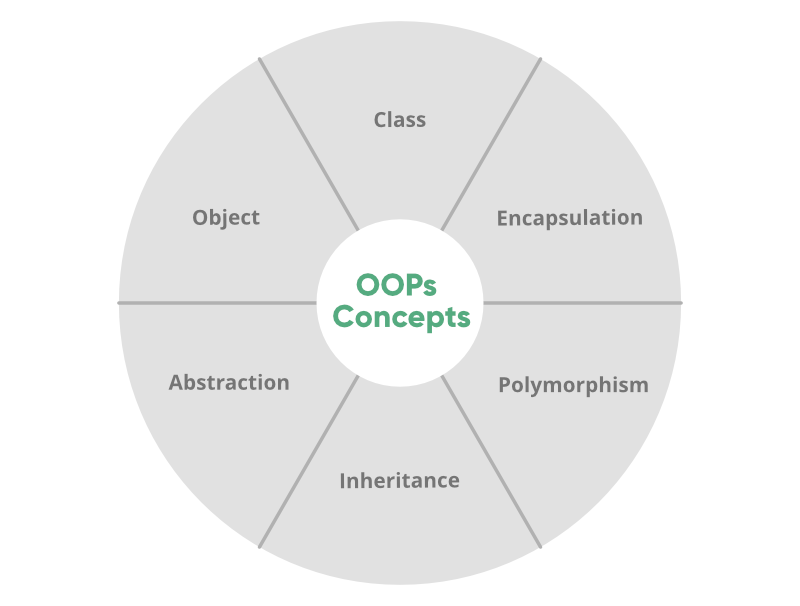Creation of a Class and an Object:
Classes and Objects are basic concepts of Object Oriented Programming which revolve around the real-life entities. A class is a user-defined blueprint or prototype from which objects are created.

## Scala

 `// A Scala program to illustrate``// how to create a class``  ` `// Name of the class is Smartphone``class` `Smartphone``{``      ` `    ``// Class variables``    ``var` `number``:` `Int ``=` `16``    ``var` `nameofcompany``:` `String ``=` `"Apple"``      ` `    ``// Class method``    ``def` `Display()``    ``{``        ``println(``"Name of the company : "` `+ nameofcompany);``        ``println(``"Total number of Smartphone generation: "` `+ number);``    ``}``}``object` `Main ``{``      ` `    ``// Main method``    ``def` `main(args``:` `Array[String]) ``    ``{``          ` `        ``// Class object``        ``var` `obj ``=` `new` `Smartphone();``        ``obj.Display();``    ``}``}`

Output:

```Name of the company : Apple
Total number of Smartphone generation: 16```

Traits

Traits are like interfaces in Java. But they are more powerful than the interface in Java because in the traits you are allowed to implement the members. Traits can have methods(both abstract and non-abstract), and fields as its members.
Creating a trait –

## Scala

 `// Scala program to illustrate how to ``// create traits``  ` `// Trait ``trait` `MyTrait``{``    ``def` `pet ``    ``def` `pet``_``color``}``  ` `// MyClass inherits trait``class` `MyClass ``extends` `MyTrait``{``      ` `    ``// Implementation of methods of MyTrait``    ``def` `pet()``    ``{``        ``println(``"Pet: Dog"``)``    ``}``      ` `    ``def` `pet``_``color()``    ``{``        ``println(``"Pet_color: White"``)``    ``}``      ` `    ``// Class method``    ``def` `pet``_``name()``    ``{``        ``println(``"Pet_name: Dollar"``)``    ``}``} ``  ` `object` `Main ``{``      ` `    ``// Main method``    ``def` `main(args``:` `Array[String]) ``    ``{``        ``val` `obj ``=` `new` `MyClass();``        ``obj.pet();``        ``obj.pet``_``color();``        ``obj.pet``_``name();``    ``}``}`

Output :

```Pet: Dog
Pet_color: White
Pet_name: Dollar```

Regular Expression

Regular Expressions explain a common pattern utilized to match a series of input data so, it is helpful in Pattern Matching in numerous programming languages. In Scala Regular Expressions are generally termed as Scala Regex.

## Scala

 `// Scala program for Regular``// Expressions` `// Creating object``object` `GfG``{` `    ``// Main method``    ``def` `main(args``             ``:` `Array[String])``    ``{` `        ``// Applying r() method``        ``val` `portal ``=` `"GeeksforGeeks"``.r``                         ``val` `CS``            ``=` `"GeeksforGeeks is a CS portal."` `            ``// Displays the first match``            ``println(portal findFirstIn CS)``    ``}``}`

Output :

`Some(GeeksforGeeks)`

To know more about tuple please refer to Regular Expressions in Scala.

Exceptions

An exception is an unwanted or unexpected event, which occurs during the execution of a program i.e at run time. These events change the flow control of the program in execution.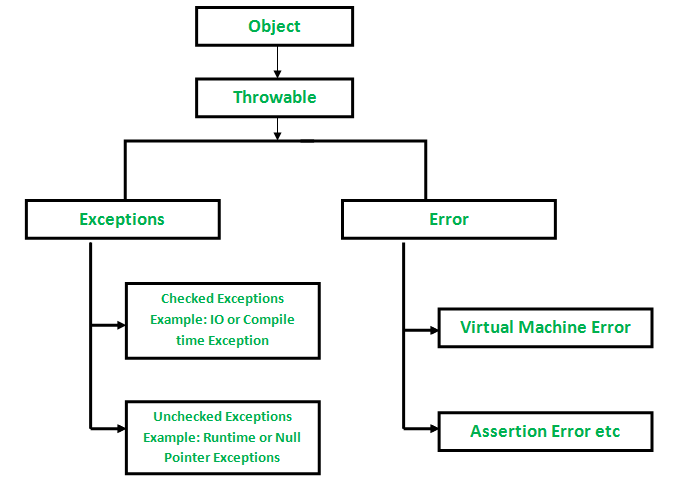In scala, All exceptions are unchecked. there is no concept of checked exception Scala facilitates a great deal of flexibility in terms of the ability to choose whether to catch an exception.
The Throwing Exceptions :

## Scala

 `// Scala program of throw keyword``  ` `// Creating object``object` `Main ``{``    ``// Define function ``    ``def` `validate(article``:``Int)``=``    ``{  ``        ``// Using throw keyword``        ``if``(article ``<` `20``)  ``            ``throw` `new` `ArithmeticException(``"You are not eligible for internship"``)  ``        ``else` `println(``"You are eligible for internship"``)  ``    ``}  ``      ` `    ``// Main method``    ``def` `main(args``:` `Array[String])``    ``{``            ``validate(``22``)``    ``}``}`

Output :

`You are eligible for internship`

Try-Catch Exceptions :

## Scala

 `// Scala program of try-catch Exception ``import` `java.io.IOException``  ` `// Creating object``object` `GFG``{  ``    ``// Main method``    ``def` `main(args``:``Array[String])``    ``{  ``        ``try``        ``{``            ``var` `N ``=` `5``/``0``               ` `        ``} ``        ``catch``        ``{``            ``// Catch block contain cases. ``            ``case` `i``:` `IOException ``=>``            ``{``                ``println(``"IOException occurred."``)``            ``}``            ``case` `a ``:` `ArithmeticException ``=>``            ``{``                ``println(``"Arithmetic Exception occurred."``)``            ``}``   ` `        ``}``   ` `    ``}  ``}  `

Output :

`Arithmetic Exception occurred.`

File Handling

File Handling is a way to store the fetched information in a file. Scala provides packages from which we can create, open, read and write the files. For writing to a file in scala we borrow java.io._ from Java because we don’t have a class to write into a file, in the Scala standard library. We could also import java.io.File and java.io.PrintWriter.

• Creating a new file :
1. java.io.File defines classes and interfaces for the JVM access files, file systems and attributes.
2. File(String pathname) converts theparameter string to abstract path name, creating a new file instance.
• Writing to the file :
java.io.PrintWriter includes all the printing methods included in PrintStream.
Below is the implementation for creating a new file and writing into it.

## Scala

 `// Scala File handling program``import` `java.io.File``import` `java.io.PrintWriter``  ` `// Creating object``object` `Geeks``{``    ``// Main method``    ``def` `main(args``:``Array[String])``    ``{``        ``// Creating a file ``        ``val` `file``_``Object ``=` `new` `File(``"abc.txt"` `) ``   ` `        ``// Passing reference of file to the printwriter     ``        ``val` `print``_``Writer ``=` `new` `PrintWriter(file``_``Object) ``  ` `        ``// Writing to the file       ``        ``print``_``Writer.write(``"Hello, This is Geeks For Geeks"``) ``  ` `        ``// Closing printwriter    ``        ``print``_``Writer.close()       ``}``}     `

•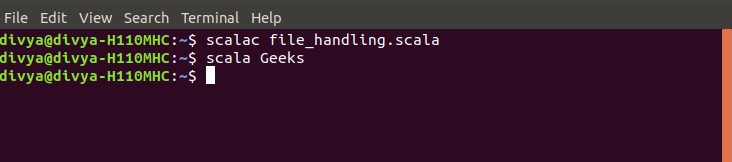Below is the example to reading a file.

## Scala

 `// Scala File handling program``import` `scala.io.Source``  ` `// Creating object ``object` `GeeksScala``{``    ``// Main method``    ``def` `main(args ``:` `Array[String])``    ``{``        ``// file name``        ``val` `fname ``=` `"abc.txt"``  ` `        ``// creates iterable representation ``        ``// of the source file            ``        ``val` `fSource ``=` `Source.fromFile(fname) ``        ``while` `(fSource.hasNext)``        ``{``            ``println(fSource.next)``        ``}``  ` `        ``// closing file``        ``fSource.close() ``    ``}``}`

•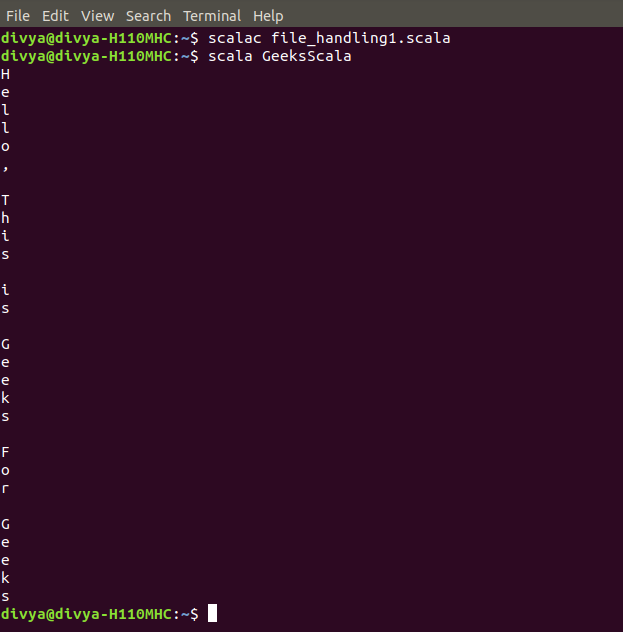To know more about various different File Handling, please refer to File Handling in Scala

Scala Collection

List

A list is a collection which contains immutable data. List represents linked list in Scala. The Scala List class holds a sequenced, linear list of items. Lists are immutable whereas arrays are mutable in Scala. In a Scala list, each element must be of the same type. list is defined under scala.collection.immutable package.
Syntax :

```val variable_name: List[type] = List(item1, item2, item3)
or
val variable_name = List(item1, item2, item3)```

Create and initialize Scala List
Example :

## Scala

 `// Scala program to print immutable lists``import` `scala.collection.immutable.``_``  ` `// Creating object``object` `GFG``{``    ``// Main method``    ``def` `main(args``:``Array[String])``    ``{  ``        ``// Creating and initializing immutable lists``        ``val` `mylist``1``:` `List[String] ``=` `List(``"Geeks"``, ``"GFG"``,``                            ``"GeeksforGeeks"``, ``"Geek123"``)``        ``val` `mylist``2` `=` `List(``"C"``, ``"C#"``, ``"Java"``, ``"Scala"``,``                                        ``"PHP"``, ``"Ruby"``)``  ` `        ``// Display the value of mylist1``        ``println(``"List 1:"``)``        ``println(mylist``1``)``  ` `        ``// Display the value of mylist2 using for loop``        ``println(``"\nList 2:"``)``        ``for``(mylist``<``-mylist``2``)``        ``{``            ``println(mylist)``        ``}``    ``}``}`

Output :

```List 1:
List(Geeks, GFG, GeeksforGeeks, Geek123)

List 2:
C
C#
Java
Scala
PHP
Ruby```

Map

Map is a collection of key-value pairs. In other words, it is similar to dictionary. Keys are always unique while values need not be unique. In order to use mutable Map, we must import scala.collection.mutable.Map class explicitly.
Creating a map and accessing the value
Example :

## Scala

 `// Scala map program of ``// Accessing Values Using Keys``  ` `// Creating object ``object` `GFG``{``   ``// Main method``    ``def` `main(args``:``Array[String])``    ``{``  ` `        ``val` `mapIm ``=` `Map(``"Ajay"` `-``>` `30``, ``                        ``"Bhavesh"` `-``>` `20``,``                        ``"Charlie"` `-``>` `50``)``  ` `        ``// Accessing score of Ajay``        ``val` `ajay ``=` `mapIm(``"Ajay"``) ``        ``println(ajay)``    ``}``} `

Output :

`30`

Iterator

An iterator is a way to access elements of a collection one-by-one. It resembles to a collection in terms of syntax but works differently in terms of functionality. To access elements we can make use of hasNext() to check if there are elements available and next() to print the next element.
Syntax:

```val v = Iterator(5, 1, 2, 3, 6, 4)

//checking for availability of next element
while(v.hasNext)

//printing the element
println(v.next)```

Example :

## Scala

 `//Scala iterator program``//for defining iterator``  ` `//Creating object``object` `GFG``{``    ``// Main method``    ``def` `main(args``:``Array[String])``    ``{``        ``val` `v ``=` `Array(``5``, ``1``, ``2``, ``3``, ``6``, ``4``)``        ``//val v = List(5, 1, 2, 3, 6, 4)``      ` `        ``// defining an iterator``        ``// for a collection``        ``val` `i ``=` `v.iterator``      ` `        ``while` `(i.hasNext)``            ``print(i.next + ``" "``)``    ``}``}`

Output:

`5 1 2 3 6 4 `

Set

A set is a collection which only contains unique items. The uniqueness of a set are defined by the == method of the type that set holds. If you try to add a duplicate item in the set, then set quietly discard your request.
Syntax :

```// Immutable set
val variable_name: Set[type] = Set(item1, item2, item3)
or
val variable_name = Set(item1, item2, item3)

// Mutable Set
var variable_name: Set[type] = Set(item1, item2, item3)
or
var variable_name = Set(item1, item2, item3)```

Creating and initializing Immutable set
Example :

## Scala

 `// Scala program to illustrate the ``// use of immutable set``import` `scala.collection.immutable.``_``  ` `object` `Main ``{``    ``def` `main(args``:` `Array[String]) ``    ``{``          ` `        ``// Creating and initializing immutable sets``        ``val` `myset``1``:` `Set[String] ``=` `Set(``"Geeks"``, ``"GFG"``, ``                            ``"GeeksforGeeks"``, ``"Geek123"``)``        ``val` `myset``2` `=` `Set(``"C"``, ``"C#"``, ``"Java"``, ``"Scala"``, ``                                          ``"PHP"``, ``"Ruby"``)``          ` `        ``// Display the value of myset1``        ``println(``"Set 1:"``)``        ``println(myset``1``)``          ` `        ``// Display the value of myset2 using for loop``        ``println(``"\nSet 2:"``)``        ``for``(myset``<``-myset``2``)``        ``{``            ``println(myset)``        ``}``    ``}``}`

Output :

```Set 1:
Set(Geeks, GFG, GeeksforGeeks, Geek123)

Set 2:
Scala
C#
Ruby
PHP
C
Java```

Creating and initializing mutable set
Example :

## Scala

 `// Scala program to illustrate the ``// use of mutable set``import` `scala.collection.immutable.``_``  ` `object` `Main ``{``    ``def` `main(args``:` `Array[String])``    ``{``          ` `        ``// Creating and initializing mutable sets``        ``var` `myset``1``:` `Set[String] ``=` `Set(``"Geeks"``, ``"GFG"``, ``                            ``"GeeksforGeeks"``, ``"Geek123"``)``        ``var` `myset``2` `=` `Set(``10``, ``100``, ``1000``, ``10000``, ``100000``)``          ` `        ``// Display the value of myset1``        ``println(``"Set 1:"``)``        ``println(myset``1``)``          ` `        ``// Display the value of myset2 ``        ``// using a foreach loop``        ``println(``"\nSet 2:"``)``        ``myset``2``.foreach((item``:``Int)``=>``println(item))``    ``}``}`

Output :

```Set 1:
Set(Geeks, GFG, GeeksforGeeks, Geek123)

Set 2:
10
100000
10000
1000
100```

To know more about Set please refer to Set in Scala | Set-1 and Set in Scala | Set-2.

Tuple

Tuple is a collection of elements. Tuples are heterogeneous data structures, i.e., is they can store elements of different data types. A tuple is immutable, unlike an array in scala which is mutable.
Creating a tuple and accessing an element
Example :

## Scala

 `// Scala program to access ``// element using underscore method``  ` `// Creating object``object` `gfg ``{``    ``// Main method``    ``def` `main(args``:` `Array[String]) ``    ``{``  ` `        ``var` `name ``=` `(``15``, ``"chandan"``, ``true``)``  ` `        ``println(name.``_``1``) ``// print 1st element``        ``println(name.``_``2``) ``// print 2st element``        ``println(name.``_``3``) ``// print 3st element``    ``}``}`

Output :

```15
chandan
true```